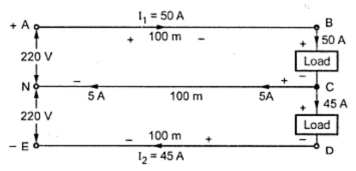### D.C. Three Wire System

It is known that higher the voltage level, lower are the transmission losses. But in case of d.c. distribution, level cannot be increased readily like a.c. Using rotating machinery, the d.c. voltage level can be increased but the method is too expensive. The d.c. three wire system can be used to double the transmission voltage, without increasing the voltage between either conductor and earth. The higher voltage demand also can be satisfied using d.c. three wire distribution system.

In this system, two generators are connected in series, each is generating a voltage of V volts. The common point is neutral from where neutral wire is run. The voltage V of each generator is with respect to neutral which is earthed. Thus the voltage between each line and neutral is V volts while between the lines it is 2 V volts. Thus the consumers demanding higher voltage are connected to the two lines while the consumers demanding less voltage for lighting load are connected to the two lines while the consumers demanding less voltage for lighting load are connected between any one line and neutral. The Fig. 1 shows the d.c. three wire distribution system.Fig. 1 Three wire d.c. system

The light loads, domestic loads are connected between any of the two lines and neutral while the d.c. motor loads requiring higher voltage are connected between the lines. The neutral is earthed.
The symbolic representation if three wire d.c. system is shown in the Fig. 2.Fig. 2 Three wire d.c. system with balanced loads

The Fig. 2 shows the current distribution in the system. The one line carries current I1 while the other current carries current I1. When the load is balanced, that is load connected on either sides of the neutral wire is exactly half of the potential between the two outer lines. Thus the positive outer wire is at V volts above the neutral while the negative outer is at V volts below the neutral.
If the load are not balanced then the neutral carries the current. This current is the difference between the two line currents I1 and Iand is called out of balance current. If the load on the positive line i.e. I1 is greater than I2 then neutral wire carries current equal to I1 - I2 if the load on the negative line is greater i.e. I2 is greater than I1 then the neutral wire carries current equal to II1. This is shown in the Fig. 3(a) and (b). The direction of I1 - I2 is from load end to supply end while the direction of I2 - I1  is from supply end to load end.Fig. 3 Out of balance current through neutral

In any of the two cases of out of balance current conditions, the neutral potential will not remain half of that between the two lines.
Instead of using two generators in series, a single generator having twice the line to neutral voltage rating also can be used.
This is shown in the Fig. 4.
In such system, two small d.c. machines are connected across the lines in series which are mechanically coupled to a common shaft. These are called balancers.
Normally when the load is balanced, machines work as the d.c. motors. In case of any out of balance current through neutral then the machine connected to lightly loaded side acts as motor while that connected to heavily loaded side as machine as motor drives the machine as generator. Thus the unbalance is compensated.Fig. 4 Use of single generator in 3 wire d.c. system
The perfect balancing can not be obtained because the working of the balancers are based on slight unbalancing of the voltages on the two sides.
1.1 Current Distribution In Three Wire D.C. System
The Fig. 5 shows 400/200 V, three wire d.c. distribution system. The total current distribution can be understood by taking concrete values of load currents. The motor load connected across the lines demand 175 A while other loads requiring less voltage, are connected between line and neutral, on both the sides of neutral. The two loads connected between positive line and neutral take 35 and 25 A current respectively while the two loads connected between negative line and neutral take 50 A current each. Applying Kirchhoff's current law at various nodes, the current in all the section can be determined as shown in the Fig. 5.Fig. 5  Current distribution

It can be seen that for the selected values, I1 = 325 A while I2  = 275 A.
Thus I2 - I1  = 275 A - 235 A = 40 A current flows at the neutral point and its direction is from neutral and towards load end. Thus knowing currents in all the sections and resistance of all the sections, the voltage across any load can be determined by applying Kirchhoff's voltage law to the appropriate loop. Such a voltage is called load point voltage. While applying Kirchhoff's voltage law, care must be taken to consider the sign of the voltage drop correctly. If the potential across two points is traced from positive to negative as a drop then it must be taken as negative while if the potential is traced from negative to positive i.e. as a rise it must be taken as positive.

Example : A three wire d.c. system takes a current of 50 A on positive side and 45 A on negative side, the resistance of each outer is 0.0004 Ω per metre while the cross-section of middle wire is half of that of each outer. If the voltage between each outer and middle wire is maintained at 220 V at the feeding end, calculate the voltage at the distant load end between each outer and middle wire. The three wires are of 100 m length.
Solution : The system is shown in the Fig. 6.Fig. 6

Now I1  = 50 A and  I2  = 45 A hence current through neutral wire is I1 - I2  = 5 A from load side to supply side.

Applying KVL to the loop ABCNA and taking potential drop as -ve and rise as + ve we can wrire,

Applying KVL to the loop NCDEN,

#### 1 comment:

1.